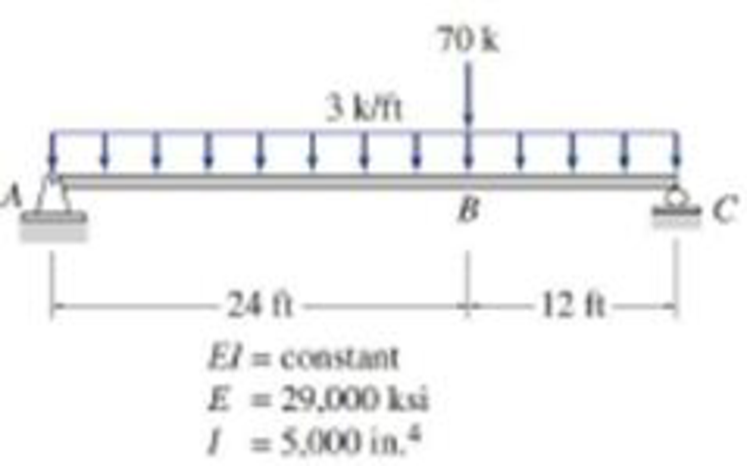# 6.49 through 6.56 Determine the maximum deflection for the beams shown in Figs. P6.23 through P6.30 by the conjugate-beam method. FIG. P6.29, P6.55

#### Solutions

Chapter
Section
Chapter 6, Problem 54P
Textbook Problem
19 views

## 6.49 through 6.56 Determine the maximum deflection for the beams shown in Figs. P6.23 through P6.30 by the conjugate-beam method.FIG. P6.29, P6.55

To determine

Find the maximum deflection Δmax for the given beam by using the conjugate-beam method.

### Explanation of Solution

Given information:

The Young’s modulus (E) is 29,000 ksi.

The moment of inertia (I) is 5,000in.4.

Calculation:

Consider flexural rigidity (EI) of the beam is constant.

Show the free body diagram of the given beam as in Figure (1).

Consider upward is positive and downward is negative.

Consider clockwise is negative and counterclowise is positive.

The given beam carries uniformly distributed load and a point load. The loading of a beam has to be divided into carrying a point load and uniformly distributed load respectively to draw the M/EI diagram.

Determine the reaction at A using the relation;

MC=0RA×36(3×36×362)=0RA=1,94436RA=54kips

Determine the support reaction at C using the relation;

V=0RA+RC(3×36)=0RC=10854RC=54kips

Show the reactions (due to uniformly distributed load) of the given beam as in Figure (2).

Determine the bending moment at A;

MA=(54×36)(3×36×362)=0

Determine the bending moment at center;

Mcenter=(3×18×182)+(54×18)=486+972=486kips-ft

Determine the bending moment at C;

MC=(54×36)(3×36×362)=1,9441,944=0

Show the M/EI diagram for the given beam as in Figure (3).

Show the free body diagram of the given beam as in Figure (4).

Determine the reaction at A using the relation;

MC=0RA×36(70×12)=0RA=84036RA=23.333kips

Determine the support reaction at C using the relation;

V=0RA+RC70=0RC=7023.333RC=46.667kips

Show the reactions as in Figure (5).

Determine the bending moment at A;

MA=(46.667×36)(70×24)=1,6801,680=0

Determine the bending moment at B;

MB=(23.333×24)=560kips-ft

Determine the bending moment at C;

MC=(23.333×36)(70×12)=840840=0

Show the M/EI diagram for the given beam as in Figure (6).

Show the conjugate beam M/EI (uniformly distributed load) diagram as in Figure (7).

Determine the conjugate beam reaction at support A and C;

RA=RC=13×(486EI)×36=5,832kips-ftEI=5,832kips-ftEI()

Show the conjugate beam M/EI (concentrated load) diagram as in Figure (8).

Determine the conjugate support reaction at support A;

MC=0RA×36+(12×36×560EI×23×24)=0RA=161,280kips-ft36EIRA=4,480kips-ftEI()

The maximum deflection will occur for the given beam at point M and take a distance of xM from point A then the slope at point M is equal to the slope at point A.

Show the section xM (uniformly distributed load) and distance xM as in Figure (9).

Refer Figure (9),

Determine the intensity of load at xM using the relation;

wM=54xM3×xM×xM2=54xM1.5xM2

Show point M (Concentrated load) and distance xM as in Figure (10).

Refer Figure (10),

The maximum deflection will occur for the given beam at point M and take a distance of xM from point A then the slope at point M is equal to the slope at point A

### Still sussing out bartleby?

Check out a sample textbook solution.

See a sample solution

#### The Solution to Your Study Problems

Bartleby provides explanations to thousands of textbook problems written by our experts, many with advanced degrees!

Get Started

Find more solutions based on key concepts
Determine the natural frequency of a pendulum whose length is 15 ft.

Engineering Fundamentals: An Introduction to Engineering (MindTap Course List)

When you are finished using a USB flash drive, simply remove it from the USB port. (383)

Enhanced Discovering Computers 2017 (Shelly Cashman Series) (MindTap Course List)

Convert P = 5.00 atm into Pa, bar, and psia.

Fundamentals of Chemical Engineering Thermodynamics (MindTap Course List)

If your motherboard supports ECC DDR3 memory, can you substitute non-ECC DDR3 memory?

A+ Guide to Hardware (Standalone Book) (MindTap Course List)